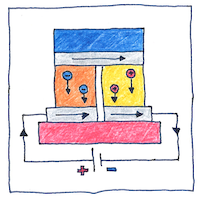# 1834 Peltier effect

## The book of science

Tom Sharp

 Jean Charles Athanase Peltier electromagnetism## Peltier effect

• Jean Charles Athanase Peltier
• put a battery on a Seebeck circuit,
• that is, an electric circuit having
• a junction of dissimilar metals,
• and found that one side of the junction
• heated up and the other side cooled.

## Findings

• William Gilbert found that the earth was a giant magnet.
• Robert Boyle found that electrical attraction would span a vacuum.
• Ewald Georg von Kleist and Pieter van Musschenbroek
• found a way to store electricity in jars.
• Benjamin Franklin found the difference
• between positive and negative electric charges.
• Charles Augustin de Coulomb found that attraction of charged objects
• depends on the amount of charge and the distance between them.
• Alessandro Volta invented a battery to produce electricity.
• Seebeck found the Seebeck effect—a temperature difference
• across different metals in a circuit created a magnetic field.
• André-Marie Ampère found that two parallel wires carrying electric currents
• attract or repel each other depending on the direction of the flow.
• Hans Christian Ørsted found a current created a magnetic field.
• Ohm found the voltage across a conductor divided by the current equals the resistance.

## Electrical units

• The ampere or amp
• the unit of current
• honors André-Marie Ampère
• who discovered the relation
• The coulomb
• the unit of charge
• honors Charles-Augustin de Coulomb
• who developed the law of electrostatic force
• of attraction and repulsion.
• the unit of capacitance
• who discovered electromagnetic induction.
• The gauss
• the unit of magnetic field strength
• honors Carl Friedrich Gauss
• who related magnetism to mass, charge, and time.
• The hertz
• the unit of frequency
• honors Heinrich Hertz
• who proved the existence of electromagnetic waves
• The henry
• the unit of inductance
• honors Joseph Henry
• who discovered electromagnetic induction
• The joule
• the unit of energy
• honors James Prescott Joule
• who discovered the relationship
• between heat and mechanical work.
• The ohm
• the unit of resistance
• honors Georg Ohm
• who discovered Ohm’s law.
• The tesla
• the unit of magnetic flux density
• honors Nikola Tesla
• who helped develop alternating current.
• The volt
• the unit of voltage
• honors Alessandro Volta
• who invented the electric battery.
• The watt
• the unit of power
• honors James Watt
• who improved the steam engine.
• The weber
• the unit of magnetic flux
• honors Wilhelm Eduard Weber
• who worked with Hertz and developed
• a system of measurements for electric currents.

## Solid-state effects

• The transistor was invented
• five years before I was born
• followed by the transistor radio when I was two.
• I found out about solid-state heating and cooling
• and solid-state generation of electricity
• when I was in the ninth grade.